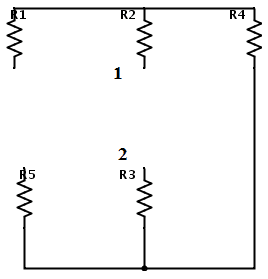# Effective resistance for Thevenin's theorem

## Homework Statement

Me again. I again got confused with 2 similar things done differently on two different places. Let's say we have some arbitrary circuit and we want to replace a part of it with thevenin's generator. We also need to calculate effective Thevenin's resistance, which is where i found the problem.Suppose we need to find the effective resistance between points 1 and 2 at the picture. First of all , what does "effective resistance between two points" even mean. Second, I would say "Rt = R2+R3+R4" and according to my workbook that is correct. But if we take a look at this example (at 3:50 for example), we will see that the "hanging" edge is also taken into account. Why ?

## Homework Equations

Thevenin's theorem

## The Attempt at a Solution

Like i mentioned, I simply used "Rt = R2+R3+R4" which turns out to be correct. Why does the guy in the video take that other edge into account??[/B]

gneill
Mentor
The effective resistance between two points means, if you were to put an Ohmmeter across those points, that's the resistance that it would measure.

I didn't watch the video, but it would appear from the still shot that the "hanging edge" you refer to is in the path of the imaginary Ohmmeter that you would use to measure the effective resistance you're looking for.

Oh. well thanks, I feel like an idiot now :D

gneill
Mentor
Oh. well thanks, I feel like an idiot now :D
:) Don't. Better to ask and banish all doubt than to leave it to muddy your thought processes forever!